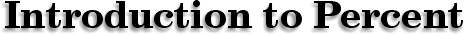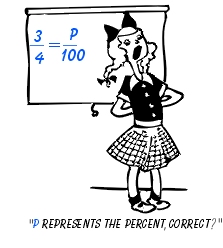#### Percent literally means "out of 100."

If a student gets 75 percent on a test or quiz, this is the equivalent of receiving 75 questions correct out of 100.We can calculate percent using proportions.

Definition: A ratio is the comparison of two measurements by division (or by a fraction bar).

A proportion is two equivalent ratios, which are written as fractions.

Example: If Betsy gets 3 problems correct out of 4, what percent did she correct?

3 = P (P represents the percent correct)
4=100

Cross multiplication will set up an equation to solve for the answer:

3 x 100 = 4 x P
300 = 4P
300
÷4 = 4P÷4

(divide each side by 4)

75 = P
Therefore, Betsy got 75 % correct.

 Example: If 150 people are surveyed and 90 people say that Bill Clinton should be able to remain president even though he may have lied under oath, what percent of the population are Clinton supporters? 90 = P 150 100 150P = 9000 9000÷150 = P P = 60%Hence, 60 % of the people think Clinton should remain as president.What percent of the cars are gray?

Converting Fractions to Percent

 mC writer:Kevin Gallagher

Percent